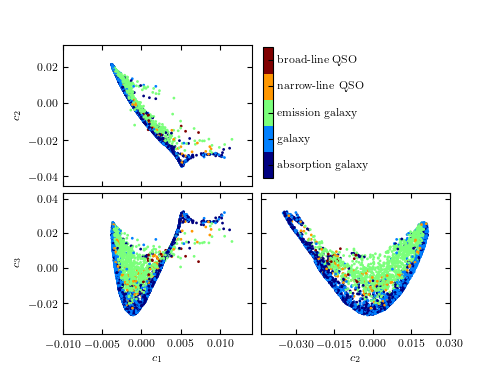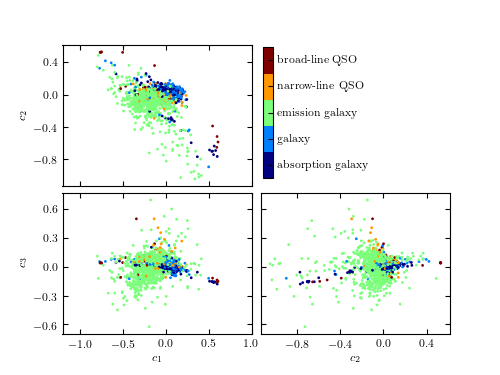# PCA Projection of SDSS Spectra¶

Figure 7.9

A comparison of the classification of quiescent galaxies and sources with strong line emission using LLE and PCA. The top panel shows the segregation of galaxy types as a function of the first three PCA components. The lower panel shows the segregation using the first three LLE dimensions. The preservation of locality in LLE enables nonlinear features within a spectrum (e.g., variation in the width of an emission line) to be captured with fewer components. This results in better segregation of spectral types with fewer dimensions.```@pickle_results: computing results and saving to 'spec_LLE.pkl'
- finished LLE projection
- removing 33 outliers for plot
```
```# Author: Jake VanderPlas
#   The figure produced by this code is published in the textbook
#   "Statistics, Data Mining, and Machine Learning in Astronomy" (2013)
#   To report a bug or issue, use the following forum:

from __future__ import print_function

import numpy as np
from matplotlib import pyplot as plt
from sklearn import manifold, neighbors

from astroML.datasets import sdss_corrected_spectra
from astroML.datasets import fetch_sdss_corrected_spectra
from astroML.plotting.tools import discretize_cmap
from astroML.utils.decorators import pickle_results

#----------------------------------------------------------------------
# This function adjusts matplotlib settings for a uniform feel in the textbook.
# Note that with usetex=True, fonts are rendered with LaTeX.  This may
# result in an error if LaTeX is not installed on your system.  In that case,
# you can set usetex to False.
if "setup_text_plots" not in globals():
from astroML.plotting import setup_text_plots
setup_text_plots(fontsize=8, usetex=True)

#------------------------------------------------------------
# Set up color-map properties
clim = (1.5, 6.5)
cmap = discretize_cmap(plt.cm.jet, 5)
cdict = ['unknown', 'star', 'absorption galaxy',
'galaxy', 'emission galaxy',
cticks = [2, 3, 4, 5, 6]
formatter = plt.FuncFormatter(lambda t, *args: cdict[int(np.round(t))])

#------------------------------------------------------------
# Fetch the data; PCA coefficients have been pre-computed
data = fetch_sdss_corrected_spectra()
coeffs_PCA = data['coeffs']
c_PCA = data['lineindex_cln']
spec = sdss_corrected_spectra.reconstruct_spectra(data)
color = data['lineindex_cln']

#------------------------------------------------------------
# Compute the LLE projection; save the results
@pickle_results("spec_LLE.pkl")
def compute_spec_LLE(n_neighbors=10, out_dim=3):
# Compute the LLE projection
LLE = manifold.LocallyLinearEmbedding(n_neighbors, out_dim,
method='modified',
eigen_solver='dense')
Y_LLE = LLE.fit_transform(spec)
print(" - finished LLE projection")

# remove outliers for the plot
BT = neighbors.BallTree(Y_LLE)
dist, ind = BT.query(Y_LLE, n_neighbors)
dist_to_n = dist[:, -1]
dist_to_n -= dist_to_n.mean()
std = np.std(dist_to_n)
flag = (dist_to_n > 0.25 * std)
print(" - removing {0} outliers for plot".format(flag.sum()))

return Y_LLE[~flag], color[~flag]

coeffs_LLE, c_LLE = compute_spec_LLE(10, 3)

#----------------------------------------------------------------------
# Plot the results:
for (c, coeffs, xlim) in zip([c_PCA, c_LLE],
[coeffs_PCA, coeffs_LLE],
[(-1.2, 1.0), (-0.01, 0.014)]):
fig = plt.figure(figsize=(5, 3.75))

# axes for colorbar
cax = plt.axes([0.525, 0.525, 0.02, 0.35])

# Create scatter-plots
scatter_kwargs = dict(s=4, lw=0, edgecolors='none', c=c, cmap=cmap)

ax1 = plt.subplot(221)
im1 = ax1.scatter(coeffs[:, 0], coeffs[:, 1], **scatter_kwargs)
im1.set_clim(clim)
ax1.set_ylabel('\$c_2\$')

ax2 = plt.subplot(223)
im2 = ax2.scatter(coeffs[:, 0], coeffs[:, 2], **scatter_kwargs)
im2.set_clim(clim)
ax2.set_xlabel('\$c_1\$')
ax2.set_ylabel('\$c_3\$')

ax3 = plt.subplot(224)
im3 = ax3.scatter(coeffs[:, 1], coeffs[:, 2], **scatter_kwargs)
im3.set_clim(clim)
ax3.set_xlabel('\$c_2\$')

fig.colorbar(im3, ax=ax3, cax=cax,
ticks=cticks,
format=formatter)

ax1.xaxis.set_major_formatter(plt.NullFormatter())
ax3.yaxis.set_major_formatter(plt.NullFormatter())

ax1.set_xlim(xlim)
ax2.set_xlim(xlim)

for ax in (ax1, ax2, ax3):
ax.xaxis.set_major_locator(plt.MaxNLocator(5))
ax.yaxis.set_major_locator(plt.MaxNLocator(5))

plt.show()
```